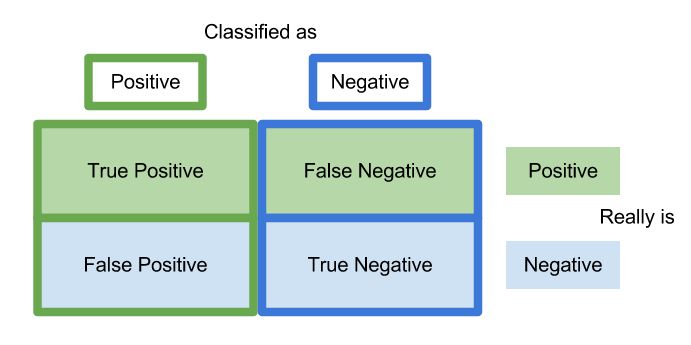# Understanding Confusion Matrices

A confusion matrix is a table that is used to organize the predictions madeduring an analysis of data. Without making a joke confusion matrices can be confusing especially for those who are new to research.

In this post, we will look at how confusion matrices are set up as well as what the information in the means.

Actual Vs Predicted Class

The most common confusion matrix is a two class matrix. This matrix compares the actual class of an example with the predicted class of the model. Below is an example

Two Class Matrix
Predicted Class
A  B
Correctly classified as A Incorrectly classified as B
Incorrectly classified as A Correctly classified as B

Actual class is along the vertical side

Looking at the table there are four possible outcomes.

• Correctly classified as A-This means that the example was a part of the A category and the model predicted it as such
• Correctly classified as B-This means that the example was a part of the B category and the model predicted it as such
• Incorrectly classified as A-This means that the example was a part of the B category but the model predicted it to be a part of the A group
• Incorrectly classified as B-This means that the example was a part of the A category but the model predicted it to be a part of the B group

These four types of classifications have four different names which are true positive, true negative, false positive, and false negative. We will look at another example to understand these four terms.

Two Class Matrix
Predicted Lazy Students
Lazy  Not Lazy
1. Correctly classified as lazy 2. Incorrectly classified as not Lazy
3. Incorrectly classified as Lazy 4. Correctly classified as not lazy

Actual class is along the vertical side

In the example above, we want to predict which students are lazy. Group one is the group in which students who are lazy are correctly classified as lazy. This is called true positive.

Group 2 are those who are lazy but are predicted as not being lazy. This is known as a false negative also known as a type II error in statistics. This is a problem because if the student is misclassified they may not get the support they need.

Group three is students who are not lazy but are classified as such. This is known as a false positive or type I error. In this example, being labeled lazy is a major headache for the students but not as dangerous perhaps as a false negative.

Lastly, group four are students who are not lazy and are correctly classified as such. This is known as a true negative.

Conclusion

The primary purpose of a confusion matrix is to display this information visually. In a future post, we will see that there is even more information found in a confusion matrix than what was cover briefly here.